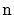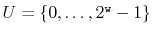5. Hash Tables

Hash tables are an efficient method of storing a small number,, of integers from a large range. The term hash table includes a broad range of data structures. This chapter focuses on one of the most common implementations of hash tables, namely hashing with chaining.

Very often hash tables store data that are not integers. In this case, an integer hash code is associated with each data item and this hash code is used in the hash table. The second part of this chapter discusses how such hash codes are generated.

Some of the methods used in this chapter require random choices of integers in some specific range. In the code samples, some of these ``random'' integers are hard-coded constants. These constants were obtained using random bits generated from atmospheric noise.

Subsections

opendatastructures.org